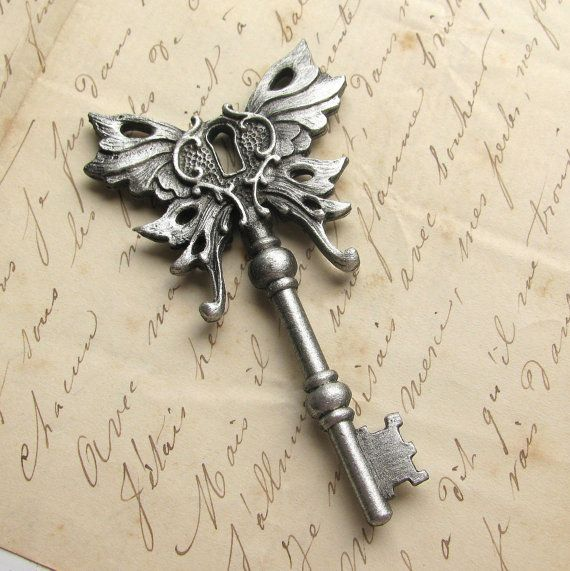# Can you crack Diffie-Hellman?Here is Eddie's take on the Diffie-Hellman Cryptosystem.

However, not using large enough key sizes can be a problem.

Given below is a generator $g$, a prime $p$ and a public key $B$ such that $g^b \equiv B \pmod p$ for some private key $b$.

Find $b$

 1 2 3 g = 4567316637081665907977181518889182113445441987446007457733573698022127469439135656733423482251931597580003871195410610463070846363265764446085651841441485; p = 20992321342930071437617535054294082600409305694823917901103517516849439468932230646876254462856426279068085286595868000559162159946064999915305764788212947; B = 13261683723811565199480160483723583184154281997005060032137595421236239575828461774398757507049515905188090075911486594228158952115852574793046073896571395; 

×